基于Vine Copula的上证股指行业板块风险度量The Risk Measurement of Shanghai Securities Industry Sectors Based on Vine Copula

• 全文下载: PDF(966KB)    PP.389-399   DOI: 10.12677/SA.2018.74045
• 下载量: 377  浏览量: 1,143   国家自然科学基金支持

After the nightmare of the 2008 financial subprime crisis, systemic risk became a major concern for policymakers and regulators. In this paper, Vine Copula is introduced to build a more flexible and accurate VaR measurement model when the traditional Copula function cannot solve the problem of “dimension disaster” better. In this paper, first of all, taking the four industry sectors’ index in Shanghai stock exchange (industrial index, commercial index, property index, utilities index) as a composite object for empirical research, the marginal distribution is fitted by using GARCH-st model. Secondly, the Pair Copula model was constructed based on different Vine-Copula structures, and the appropriate C-Vine structure Copula was selected through maximum likelihood value, AIC and BIC value. Finally, VaR from a number of portfolios is calculated by using the monte carlo simulation method, and the effectiveness of the model is tested by Kupiec return test method, in order to provide more reference for investors and risk managers.

1. 引言

2. 基本理论

2.1. Pair Copula理论

1) C的定义域： $\text{U}=\left(u,v\right)\in {\left[0,1\right]}^{2}$

2) $C\left(u,v\right)$ 有零基面且是二维递增的；

3) 对于U中的任意两点 $u,v$ ，有 $C\left(u,0\right)=0=C\left(0,v\right),C\left(u,1\right)=u,C\left(1,v\right)=v$

4) 对于U中的任意四个点 ${u}_{1},{u}_{2},{v}_{1},{v}_{2}$ ，如果 ${u}_{1}\le {u}_{2},{v}_{1}\le {v}_{2}$ ，则

$C\left({u}_{2},{u}_{2}\right)-C\left({u}_{2},{v}_{1}\right)-C\left({u}_{1},{v}_{2}\right)=C\left({u}_{1},{v}_{1}\right)\ge 0$

$F\left({x}_{1},{x}_{2}\right)=C\left({F}_{1}\left({x}_{1}\right),F\left({x}_{2}\right)\right)$ (1)

${F}_{1}\left({x}_{1}\right)$${F}_{2}\left({x}_{2}\right)$ 都是连续的，那么C是唯一存在的；反之，若 ${F}_{1}\left({x}_{1}\right)$${F}_{2}\left({x}_{2}\right)$ 是一元分布函数， $C\left({u}_{1},{u}_{2}\right)$ 为对应的Copula函数，那么(1)式定义的 $F\left({x}_{1},{x}_{2}\right)$ 函数是一个多元联合分布函数，边缘分布为 ${F}_{1}\left({x}_{1}\right)$${F}_{2}\left({x}_{2}\right)$ 。这个定理描述了Copula函数和边缘分布函数的关系，指出可以通过一个Copula连接函数将一个多维联合概率分布函数同其对应的一维边缘概率分布函数结合起来。

Aas和Czado (2009)  在文章中详细介绍了Pair-Copula的相关理论，认为多元变量联合密度函数按照某种结构可分解为一系列Pair-Copula密度函数和边缘分布函数的乘积。对于n维随机变量向量 $X=\left({x}_{1},{x}_{2},\cdots ,{x}_{n}\right)$ ，根据条件密度函数性质，X的联合密度函数可表示为

$f\left({x}_{1},{x}_{2},\cdots ,{x}_{n}\right)={f}_{n}\left({x}_{n}\right)\cdot f\left({x}_{n-1}|{x}_{n}\right)\cdot f\left({x}_{n-2}|{x}_{n-1},{x}_{n}\right)\cdot \cdots \cdot f\left({x}_{1}|{x}_{2},\cdots ,{x}_{n}\right)$ (2)

$f\left({x}_{1},{x}_{2},\cdots ,{x}_{n}\right)={c}_{12\cdots n}\left({F}_{1}\left({x}_{1}\right),{F}_{2}\left({x}_{2}\right),\cdots ,{F}_{n}\left({x}_{n}\right)\right)\cdot {f}_{1}\left({x}_{1}\right)\cdot \cdots \cdot {f}_{n}\left({x}_{n}\right)$ (3)

$f\left(x|v\right)={c}_{x{v}_{j}|{v}_{-j}}\left(F\left(x|{v}_{-j}\right),F\left({v}_{j}|{v}_{-j}\right)\right)\cdot f\left(x|{v}_{-j}\right)$ (4)

$f\left(x|v\right)=\frac{\partial {C}_{x{v}_{j}|{v}_{-j}}\left(F\left(x|{v}_{-j}\right),F\left({v}_{j}|{v}_{-j}\right)\right)}{\partial F\left({v}_{j}|{v}_{-j}\right)}$ (5)

2.2. 藤Copula理论

Vine-Copula实质是将传统的多元Copula以藤图的结构形式将多维Copula分解成一系列的二维或者条件二维Copula。正则藤(Regular Vine)最先在2001年由Bedford和Cooke  提出，后在2006年由Kurowicka和Cooke  做了详细的描述。在n元联合分布中，n维变量的藤主要是指一类树的集合，树j $\left(j=1,2,\cdots ,n-2\right)$ 的边是树 $j+1$ 的节点，每棵树的边数都取最大。其中，最特殊的两类藤是Canonical-Vine(在文中简称C-Vine)和D-Vine两种藤。C-Vine的每一棵树Tj只有单一的点连接到 $n-j$ 条边上，反观D-Vine，树中的任意一个结点最多和2条边相连。图1图2分别是四维C藤、D藤各类结构的示意图。

$f\left({x}_{1},\cdots ,{x}_{n}\right)=\underset{k=1}{\overset{n}{\prod }}f\left({x}_{k}\right)\underset{j=1}{\overset{n-1}{\prod }}\underset{i=1}{\overset{n-1}{\prod }}{c}_{j,j+i|1,\cdots ,j-1}\left(F\left({x}_{j}|{x}_{1},\cdots ,{x}_{j-1}\right),F\left({x}_{j+i}|{x}_{1},\cdots ,{x}_{j-1}\right)\right)$ (6)

$f\left({x}_{1},\cdots ,{x}_{n}\right)=\underset{k=1}{\overset{n}{\prod }}f\left({x}_{k}\right)\underset{j=1}{\overset{n-1}{\prod }}\underset{i=1}{\overset{n-1}{\prod }}{c}_{i,i+j|i+1,\cdots ,i+j-1}\left(F\left({x}_{i}|{x}_{i+1},\cdots ,{x}_{i+j-1}\right),F\left({x}_{j+i}|{x}_{i+1},\cdots ,{x}_{i+j-1}\right)\right)$ (7)

2.3. 风险度量工具VaR

VaR(Value at Risk)即在险价值，是风险测度的一个重要工具，用于表示市场正常波动在一定的置信水平下，投资者持有的证券或者资产组合在未来一定时间内可能产生的最大损失。公式表示为

$\mathrm{Pr}\left(\Delta {P}_{\Delta t}\le \text{VaR}\right)=\alpha$ (8)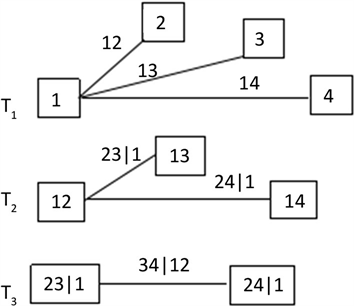Figure 1. The tree schematic diagram of C-Vine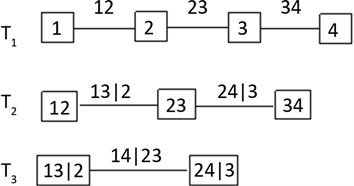Figure 2. The tree schematic diagram of D-Vine

2.4. 建模步骤

1) 求出各行业指数的日收盘价各自对应的对数收益率，并做出时序图；

2) 为了解四个收益率序列的总体情况，对其做基本描述性统计分析和平稳性检验；

3) 对收益率序列做ARCH效应检验，检验序列的异方差性，根据检验结果建立GARCH类模型；

4) 考虑t分布和偏t (skew-t，以下简称st)分布拟合序列的残差分布，并最终通过标准化GARCH-t和GARCH-st建模后的残差序列，进行概率积分变换，得到各样本的累积分布，再用K-S检验进行筛选；

5) 建立Vine-Copula模型，并通过极大似然值、AIC和BIC准则选择合适的C-Vine或D-Vine结构；

6) 利用蒙特卡罗方法计算在给定置信水平下投资组合的VaR，并做Kupiec返回检验。

3. 实证分析

3.1. 数据的选取和基本分析

${R}_{t}=\mathrm{ln}{P}_{t}-\mathrm{ln}{P}_{t-1}$ (9)

3.2. 实证研究

3.2.1. GARCH模型的选取和估计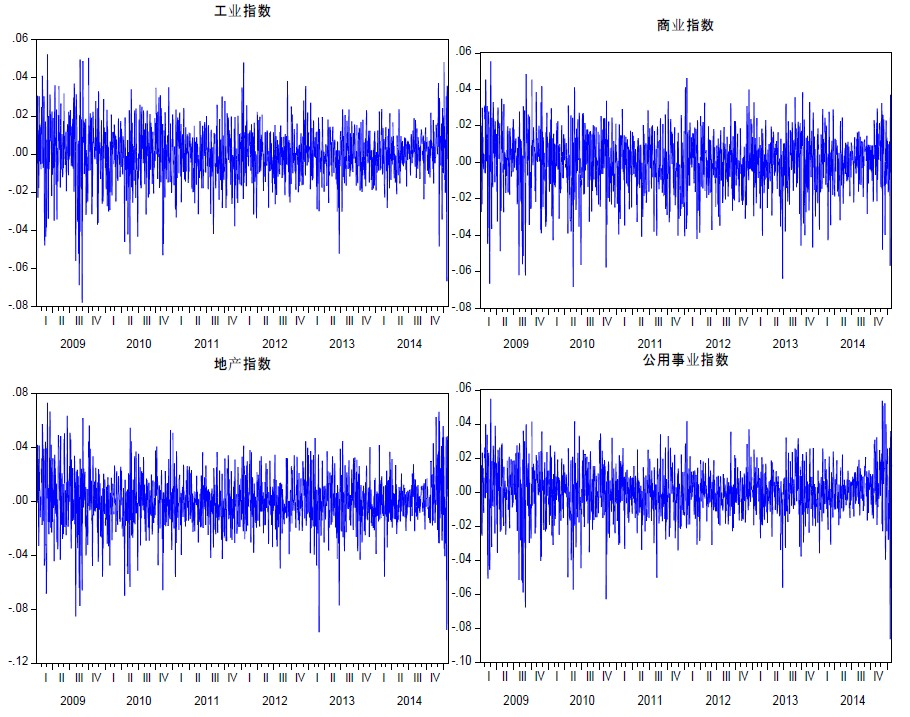Figure 3. Time series diagram of the yield rates of the four assetsTable 1. Statistical description of each sampleTable 2. Estimation results of GARCH-st model

3.2.2. [0,1]均匀分布检验

3.2.3. Vine-Copula函数建模Table 4. Kendall’s tau rank correlation coefficient of standardized residual probability integral transformation sequenceTable 5. Results of Pair-Copula fitting of rattan structures

3.2.4. VaR风险度量和模型检验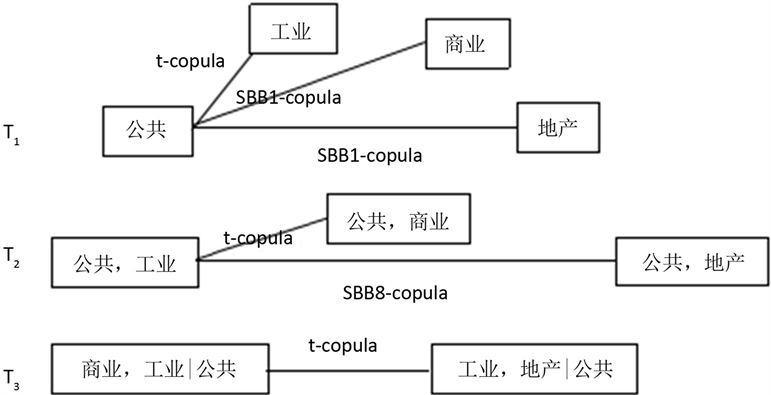Figure 4. Dependency structure diagram of C-Vine Copula model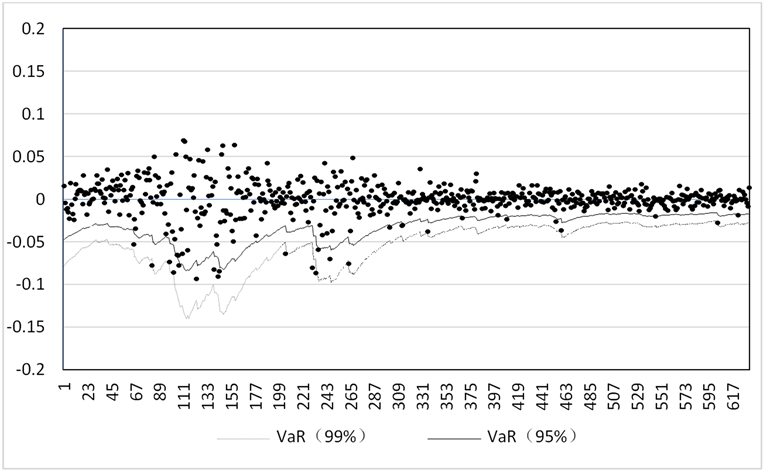Figure 5. The VaR result based on the C-Vine Copula modelTable 6. Kupeic test based on failure rate of GARCH-st-C-Vine Copula model

4. 结论

1) 工业指数、商业指数、地产指数和公用事业指数四个资产组合的对数收益率均具有“波动集聚”的特点，且为平稳序列，四个资产组合的对数收益率均具有“尖峰厚尾”的特征，并不服从正态分布，不能用正态分布来拟合其边缘分布，考虑了t分布和偏t分布，最后选择符合条件的偏t分布。

2) 在实证分析中描述了四个资产组合各自的日对数收益率序列的基本特征之后，并做了相关的检验，最终工业指数、商业指数、公用事业指数三者对数收益率序列选择GARCH(1,1)模型，地产指数对数收益率序列选择GARCH(1,2)模型。

3) 在藤Copula模型的构建中，对比C藤与D藤在四个资产组合的AIC和BIC、极大似然值，发现在工业指数、商业指数、地产指数和公用事业指数四个资产组合中，C-Vine Copula更适合。

4) 选择在偏t分布假设下的GARCH模型，结合C-Vine Copula模型，分别计算在99%、95%置信水平下的VaR，并通过Kupiec返回检验对模型进行后验测试，说明了本文模型构造的有效性，并发现了不同的置信水平下，99%下的VaR值精度更高。

  Embrechts, P., McNeil, A. and Straumann, D. (1999) Correlation: Pitfalls and Alternatives. RISK, 12, 69-71.  张尧庭. 连接函数(copula)技术与金融风险分析[J]. 统计研究, 2002, 19(4): 48-51.  叶伟, 杨招军. 基于Copula-VaR方法的外汇储备风险度量[J]. 统计与决策, 2015(3): 153-156.  鲁思瑶, 徐美萍. 基于扭曲混合Copula和ARMA-GARCH-t模型的投资组合风险分析——以上证综指、中证综合债和上证基金为例[J]. 数理统计与管理, 2017, 36(6): 1131-1140.  Bedford, T. and Cooke, R.M. (2001) Probability Density Decomposition for Conditionally Dependent Random Variables Modeled by Vines. Annals of Ma-thematics and Artificial Intelligence, 32, 245-268.  Bedford, T. and Cooke, R.M. (2002) Vines—A New Graphical Model for Dependent Random Variables. Annals of Statics, 30, 1031-1068. https://doi.org/10.1214/aos/1031689016  Aas, K., Czado, C., Frigessi, A. and Bakken, H. (2009) Pair-Copula Constructions of Multiple Dependence. Insurance: Mathematics and Economics, 44, 182-198. https://doi.org/10.1016/j.insmatheco.2007.02.001  Hoffman, M. and Czado, C. (2010) Assessing the VaR of a Portfolio Using D-Vine Copula Based Multivariate GARCH Models. https://mediatum.ub.tum.de/doc/1079206/1079206.pdf  高江. 藤Copula模型与多资产投资组合VaR预测[J]. 数理统计与管理, 2013, 32(2): 247-258.  张高勋, 田益祥, 李秋敏. 基于Pair Copula模型的资产组合VaR比较研究[J]. 系统管理学报, 2013, 22(2): 223-231.  Nelsen, R.B. (1999) An Introduction to Copula. Springer, New York. https://doi.org/10.1007/978-1-4757-3076-0  Sklar, A. (1959) Fonctions de répartition à n dimen-sions et leurs marges. Publications De, l'Institut de Statistique de Paris, 8.  Kurowicka, D. and Cooke, R. (2006) Uncertainty Analysis with High Dimensional Dependence Modeling. Wiley, Chichester. https://doi.org/10.1002/0470863072  Brechmann, E.C., Schepsmeier, U., Grün, B., et al. (2013) Modeling Dependence with C- and D-Vine Copulas: The R Package CDVine. Journal of Statistical Software, 52, Issue 3. https://doi.org/10.18637/jss.v052.i03